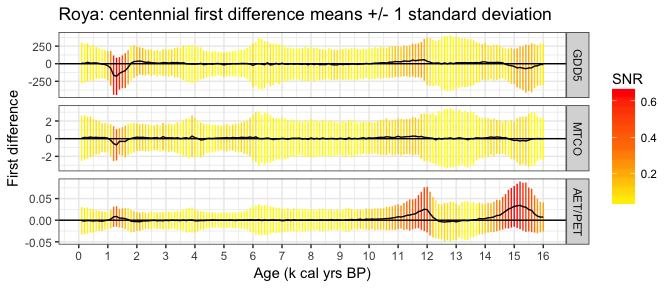# Bayesian Palaeoclimate Reconstruction from Pollen data with Bclim

## Introduction

This vignette contains the code and associated descriptions to re-create the plots given in the Parnell et al submitted paper Joint palaeoclimate reconstruction from pollen data via forward models and climate histories. It is not meant to be a completed vignette, and it is not a complete instruction manual yet. If you spot bugs or mistakes please post to the GitHub repository. Some of the code below takes a long time to run, so you can shortcut the steps by downloading the resulting files from my website. The places where this is relevant are all given in the code below.

## Installation

The stable version can be downloaded at the R command prompt with:

install.packages('Bclim')

The latest version, which usually contains extra bug fixes, or experimental functions which might not work well yet, is available via:

devtools::install_github('andrewcparnell/Bclim')

Note that for the above you need to have installed the devtools package.

If the installation has worked correctly no error should be returned upon typing:

library(Bclim)

## Roya

To create the plots for Roya we first need to create a chronology using the Bchron package before we can run Bclim on it.

We run the Bchron step with:

library(Bchron)

"Roya.dat",
package = "Bclim"),

# Load in the pollen depths
"Roya_pollen_depths.txt",
package = "Bclim"))

# Run Bchron
Roya_chron = with(Roya_dates,
Bchronology(ages=Age,
ageSds=sd,
positions=Depth,
positionThickness=Thickness,
id=id,
predictPositions=Roya_depths$V1, thin = 4)) We can check convergence with: # Check convergence summary(Roya_chron,type='convergence') # Good ## Convergence check (watch for too many small p-values): ## p-value ## Roya_6 0.01487 ## Outlier 3 0.02045 ## RateMean 0.06030 ## Outlier 2 0.07437 ## Roya_3 0.08490 ## Roya_5 0.15432 ## Outlier 5 0.16415 ## Roya_2 0.20382 ## Outlier 1 0.24442 ## Outlier 4 0.25081 ## Outlier 6 0.34662 ## RateVar 0.35740 ## Roya_1 0.42724 ## Roya_4 0.47863 And plot the chronology with # Plot plot(Roya_chron,xlab='Age (cal BP)',ylab='Depth (cm)',main='Laguna de la Roya',las=1)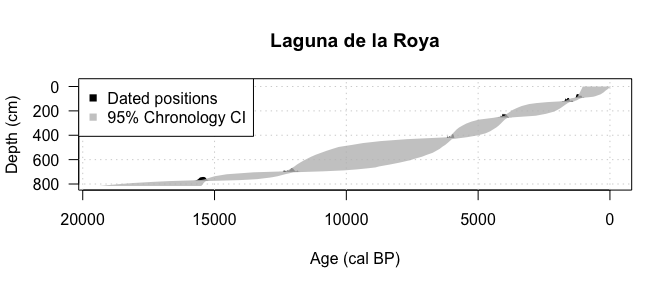We can now run Bclim with: # Load in the pollen data Roya_pollen = read.table(system.file("extdata", "Roya_pollen.txt", package = "Bclim"), header=TRUE) # Extract the chronologies - Bclim requires them in thousands of years Roya_chronologies = Roya_chron$thetaPredict/1000

# Run the single slice climate clouds
Roya_slices = slice_clouds(Roya_pollen)

# Run the climate histories function
Roya_histories = climate_histories(slice_clouds = Roya_slices,
chronology = Roya_chronologies,
time_grid = seq(0,16,by=0.1))

We can plot a single slice of the climate clouds:

# Plot a single slice cloud if required - plot slice 50
par(mar=c(3,3,2,1), mgp=c(2,.7,0), tck=-.01,las=1)
plot(Roya_histories$slice_clouds,slice=50, dims=2:3, main='slice 50',xlab='MTCO',ylab='AET/PET',col=terrain.colors(30))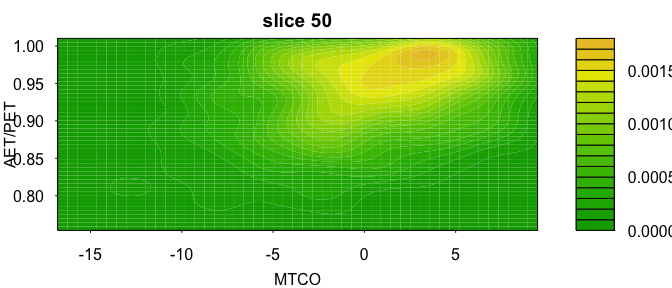Plot the climate histories: # Plot the climate histories par(mar=c(3,3,2,1), mgp=c(2,.7,0), tck=-.01,las=1) plot(Roya_histories, most_representative = 3, chron=Roya_chronologies,xlab='Age (k cal years BP)',ylab='GDD5',main='Roya GDD5') legend('topleft',legend=c('Slice clouds','Climate ribbon','Representative histories'),pch=15,col=c('blue','red','green'))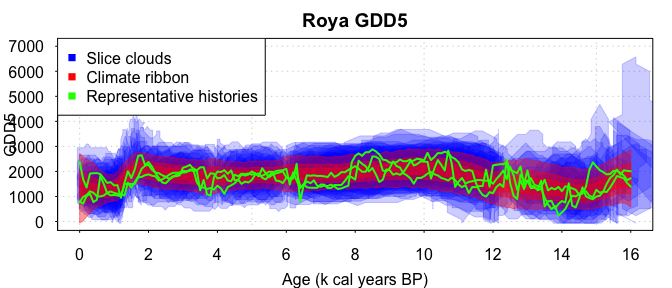plot(Roya_histories,dim=2,most_representative = 3,chron=Roya_chronologies,xlab='Age (k cal years BP)',ylab='MTCO',main='Roya MTCO') legend('bottomleft',legend=c('Slice clouds','Climate ribbon','Representative histories'),pch=15,col=c('blue','red','green'))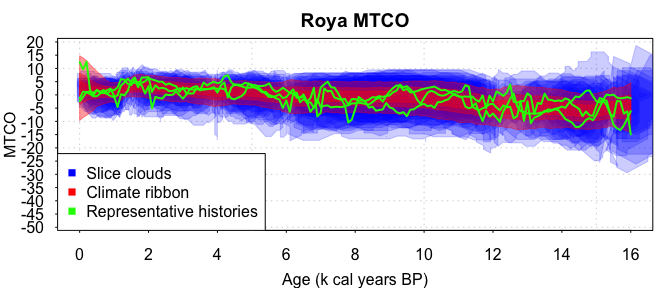par(mar=c(3,3,2,1), mgp=c(2,.7,0), tck=-.01,las=1) plot(Roya_histories,dim=3,most_representative = 3, chron=Roya_chronologies,xlab='Age (k cal years BP)',ylab='AET/PET',main='Roya AET/PET') legend('bottomleft',legend=c('Slice clouds','Climate ribbon','Representative histories'),pch=15,col=c('blue','red','green'))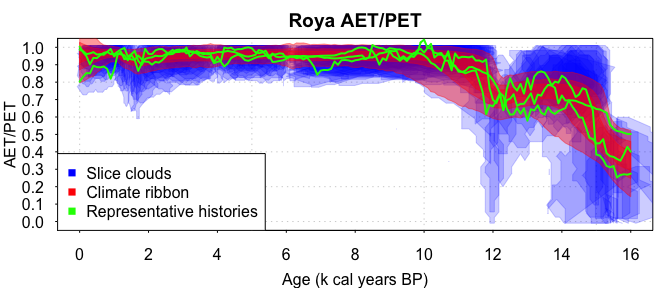We can summarise the histories with e.g. (results not shown) # Summarise the histories if required summary(Roya_histories,dim=3) And finally create the plots of the first differences: ### Create the plot of first differences # This might one day become a function in Bclim # Somewhere to store the differences diffs = vector('list',length=3) clim_names = c('GDD5','MTCO','AET/PET') # Loop through each climate dimension for(i in 1:3) { diffs[[i]] = -apply(Roya_histories$histories[,,i],1,'diff')
}
diff_means = lapply(diffs,'rowMeans')
diff_sds = lapply(diffs,function(x) apply(x,1,'sd'))
diff_snr = lapply(mapply("/",diff_means,diff_sds,SIMPLIFY = FALSE),'abs')
df = data.frame(tg=rep(Roya_histories$time_grid[-1],3), diff_means=unlist(diff_means), diff_sds=unlist(diff_sds), SNR=unlist(diff_snr), clim_var=rep(clim_names, each=length(Roya_histories$time_grid)-1))
df$clim_var = factor(df$clim_var,levels=clim_names,ordered=TRUE)

# Create the plot using ggplot2
library(ggplot2)
limits = aes(ymax = diff_means + diff_sds, ymin= diff_means - diff_sds)
p = ggplot(df,aes(x=tg,y=diff_means,colour=SNR)) +
geom_linerange(limits) +
geom_line(colour='black') +
facet_grid(clim_var ~ .,scales='free_y')+ xlab('Age (k cal yrs BP)') +
ylab('First difference') +
ggtitle('Roya: centennial first difference means +/- 1 standard deviation') +
scale_x_continuous(breaks=seq(0,16,by=1))+theme_bw() +
geom_hline(aes(yintercept=0)) +
print(p)